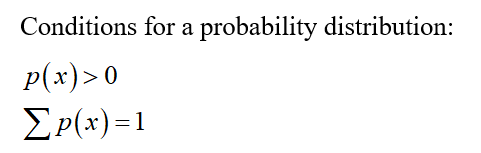# Is a probability distribution defined if the only possible values of a random variable are 0, 1, 2, 3, and P(0)=p(1)=p(2)=p(3)=1/3?

Question
1 views

Is a probability distribution defined if the only possible values of a random variable are 0, 1, 2, 3, and P(0)=p(1)=p(2)=p(3)=1/3?

check_circle

Step 1

Given that,

Step 2Step 3

### Want to see the full answer?

See Solution

#### Want to see this answer and more?

Solutions are written by subject experts who are available 24/7. Questions are typically answered within 1 hour.*

See Solution
*Response times may vary by subject and question.
Tagged in

### Statistics